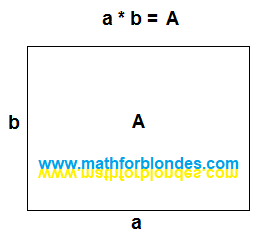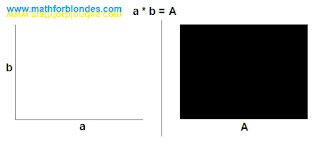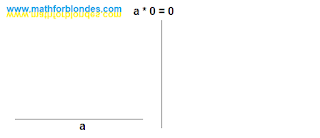## 8/11/2022

### Multiplication by zero in geometry

Consider multiplication by zero in geometry. Multiplying the length by the width gives the area. Whatever mathematicians tell you, all the formulas for the areas of any geometric shapes come down to this simple formula. It’s just that for different figures there are different correction factors, but we’ll talk about this some other time.

We have a rectangle and a formula to calculate its area. Traditionally, it is portrayed as follows.Rectangle area

Everything seems to be correct. But... In algebra, we first write down the two factors, then we write the equal sign, and only after that we write down the result of the multiplication. This is a whole series, not just one picture. Let's represent geometrically everything that we write down algebraically. The sides of the rectangle a and b are line segments. The mathematical operation of multiplication has no geometric representation. I will replace the equal sign with a vertical line that divides the picture into two parts - before multiplication and the result of multiplication. The area of rectangle A is, in fact, the rectangle itself. Here's what happened.Multiplication in geometry

Two perpendicular segments before multiplication turn into the area of a rectangle after multiplication. Now let's draw multiplication by zero.Multiplication by zero

As a result of multiplication by zero, we do not get the area. We have the length, but the width is missing. Naturally, the area is simply nowhere to come from. We look at the result of multiplying zero.Multiplying zero

Now we have a width but no length. Again, the area can not be obtained. Next, we depict the multiplication of zeros - zero multiplied by zero equals zero.Multiplication of zeros

No length, no width, no area. We take nothing, multiply by nothing and get nothing as a result. And now the most interesting.

Let's try to depict geometrically that intimate moment when we do not perform multiplications. Even algebra is bashfully silent about this. How to algebraically write down the fact that we have two segments that can be interpreted as length and width, but we do not perform the mathematical operation of multiplication between them? Not a single fool would come up with such a thing, and fools are not taught this. Sentient beings differ from trained animals in that they can do more than just what their trainers have taught them. We were taught that we perform multiplication only on the command "Milo, multiply!" or when we see the multiplication sign, we simply ignore all other cases. That's why the symbol "do not multiply" is absent in mathematics. I use "and" instead.We don't do multiplication

If we do not perform multiplication, there is no area. Now we can compare the results. If we perform multiplication, the result is an area. If we do not perform multiplication, there is no area.

Conclusion: when operating with zero, the mathematical operation of multiplication is not performed.

It is impossible to perform multiplication with zero - it will not work. It's like sawing air. You pick up a saw, move it back and forth and tell everyone on the phone that you are sawing. Only at the same time you do not specify that you are sawing something that, in principle, it is impossible to saw. By the way, we will consider the physics of multiplication by zero separately.